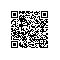# 用 Go 语言理解 Tensorflow

Tensorflow 并不是一个严格意义上的机器学习库，它是一个使用图来表示计算的通用计算库。它的核心功能由 C++ 实现，通过封装，能在各种不同的语言下运行。它的 Golang 版和 Python 版不同，Golang 版 Tensorflow 不仅能让你通过 Go 语言使用 Tensorflow，还能让你理解 Tensorflow 的底层实现。

## 封装

• C++ 源码：底层和高层的具体功能由 C ++ 源码实现，它是真正 Tensorflow 的核心。

• Python 封装与Python 库：由 C++ 实现自动生成的封装版本，通过这种方式我们可以直接用 Python 来调用 C++ 函数：这也是 numpy 的核心实现方式。

Python 库通过将 Python 封装版的各种调用结合起来，组成了各种广为人知的高层 API。

• Java 封装

• Go 封装

## Go 封装TensorFlow 为 Go 编程提供了一些 API。这些 API 特别适合加载在 Python 中创建的模型，让其在 Go 应用 中运行。

## 理解 Tensorflow 的结构

TensorFlow™ 是一个采用数据流图(data flow graphs),用于数值计算的开源软件库。节点（Nodes）在图中表示数学操作，图中的线（edges）则表示在节点间相互联系的多维数据数组，即张量（tensor）。

API 文档中明确告知了 tensorflow（简称 tf）包与 op 包中的可用方法列表。

tf 包中包含了各种构建基础结构的函数，例如 Graph（图）。op 包是最重要的包，它包含了由 C++ 实现自动生成的绑定等功能。package main

import (
"fmt"
tf "github.com/tensorflow/tensorflow/tensorflow/go"
"github.com/tensorflow/tensorflow/tensorflow/go/op"
)

func main() {
// 第一步：创建图

// 首先我们需要在 Runtime 定义两个 placeholder 进行占位
// 第一个 placeholder A 将会被一个 [2, 2] 的 interger 类型张量代替
// 第二个 placeholder x 将会被一个 [2, 1] 的 interger 类型张量代替

// 接下来我们要计算 Y = Ax

// 创建图的第一个节点：让这个空节点作为图的根
root := op.NewScope()

// 定义两个 placeholder
A := op.Placeholder(root, tf.Int64, op.PlaceholderShape(tf.MakeShape(2, 2)))
x := op.Placeholder(root, tf.Int64, op.PlaceholderShape(tf.MakeShape(2, 1)))

// 定义接受 A 与 x 输入的 op 节点
product := op.MatMul(root, A, x)

// 每次我们传递一个域给一个操作的时候，
// 我们都要将操作放在在这个域下。
// 如你所见，现在我们已经有了一个空作用域（由 newScope）创建。这个空作用域
// 是我们图的根，我们可以用“/”表示它。

// 现在让 tensorflow 按照我们的定义建立图吧。
// 依据我们定义的 scope 与 op 结合起来的抽象图，程序会创建相应的常数图。

graph, err := root.Finalize()
if err != nil {
// 如果我们错误地定义了图，我们必须手动修正相关定义，
// 任何尝试自动处理错误的方法都是无用的。

// 就像 SQL 查询一样，如果查询不是有效的语法，我们只能重写它。
panic(err.Error())
}

// 如果到这一步，说明我们的图语法上是正确的。
// 现在我们可以将它放在一个 Session 中并执行它了！

var sess *tf.Session
sess, err = tf.NewSession(graph, &tf.SessionOptions{})
if err != nil {
panic(err.Error())
}

// 为了使用 placeholder，我们需要创建传入网络的值的张量
var matrix, column *tf.Tensor

// A = [ [1, 2], [-1, -2] ]
if matrix, err = tf.NewTensor(int64{ {1, 2}, {-1, -2} }); err != nil {
panic(err.Error())
}
// x = [ ,  ]
if column, err = tf.NewTensor(int64{ {10}, {100} }); err != nil {
panic(err.Error())
}

var results []*tf.Tensor
if results, err = sess.Run(map[tf.Output]*tf.Tensor{
A: matrix,
x: column,
}, []tf.Output{product}, nil); err != nil {
panic(err.Error())
}
for _, result := range results {
fmt.Println(result.Value().([][]int64))
}
}

go run attempt1.go

panic: failed to add operation "Placeholder": Duplicate node name in graph: 'Placeholder'

## 第 1 课：node IDs

import tensorflow as tf
a = tf.placeholder(tf.int32, shape=())
b = tf.placeholder(tf.int32, shape=())
add = tf.add(a,b)
sess = tf.InteractiveSession()
print(sess.run(add, feed_dict={a: 1,b: 2}))

#### 提问时间：

• 关于 Tensorflow 的架构我们学到了什么？

图中的每个节点都必须有唯一的名称。所有节点都是通过名称进行辨认。

• 节点名称与定义操作符的名称是否相同？

是的，也可说节点名称是操作符名称的最后一段。

## 第 2 课：作用域

/// 返回新的作用域。所有在返回的作用域中的 op 都会被命名为
/// <name>/<op_name>[_<suffix].
Scope WithOpName(const string& op_name) const;

Scope 就是从根 /（空图）追溯至 op_name 的完整路径

WithOpName 方法在我们尝试添加一个有着相同的 / 到 op_name 路径的节点时，为了避免在相同作用域下有重复的节点，会为其加上一个后缀 _<suffix><suffix> 是一个计数器）。

SubScope 将会返回一个新的 Scope，这个 Scope 能确保所有的被加入图中的操作都被放置在 ‘namespace’ 的命名空间下。如果这个命名空间和作用域中已经存在的命名空间冲突，将会给它加上后缀。

A := op.Placeholder(root, tf.Int64, op.PlaceholderShape(tf.MakeShape(2, 2)))
x := op.Placeholder(root, tf.Int64, op.PlaceholderShape(tf.MakeShape(2, 1)))

// 在根定义域下定义两个自定义域，命名为 input。这样
// 我们就能在根定义域下拥有 input/ 和 input_1/ 两个定义域了。
A := op.Placeholder(root.SubScope("input"), tf.Int64, op.PlaceholderShape(tf.MakeShape(2, 2)))
x := op.Placeholder(root.SubScope("input"), tf.Int64, op.PlaceholderShape(tf.MakeShape(2, 1)))
fmt.Println(A.Op.Name(), x.Op.Name())

input/Placeholder input_1/Placeholder

#### 提问时间：

• 关于 Tensorflow 的架构我们学到了什么？

节点完全由其定义所在的作用域标识。这个”作用域“是我们从图的根节点追溯到指定节点的一条路径。有两种方法来定义执行同一种操作的节点：1、将其定义放在不同的作用域中（Go 风格）2、改变操作名称（我们在 C++ 中可以这么做，Python 版会自动这么做）

panic: failed to add operation "MatMul": Value for attr 'T' of int64 is not in the list of allowed values: half, float, double, int32, complex64, complex128

Value for attr ‘T’ of int64 is not in the list of allowed values: half, float, double, int32, complex64, complex128

## 第 3 课：Tensorflow 类型系统

REGISTER_OP("MatMul")
.Input("a: T")
.Input("b: T")
.Output("product: T")
.Attr("transpose_a: bool = false")
.Attr("transpose_b: bool = false")
.Attr("T: {half, float, double, int32, complex64, complex128}")
.SetShapeFn(shape_inference::MatMulShape)
.Doc(R"doc(
Multiply the matrix "a" by the matrix "b".
The inputs must be two-dimensional matrices and the inner dimension of
"a" (after being transposed if transpose_a is true) must match the
outer dimension of "b" (after being transposed if transposed_b is
true).
*Note*: The default kernel implementation for MatMul on GPUs uses
cublas.
transpose_a: If true, "a" is transposed before multiplication.
transpose_b: If true, "b" is transposed before multiplication.

• 名称: MatMul
• 参数: ab
• 属性（可选参数）: transpose_atranspose_b
• 模版 T 支持的类型: half, float, double, int32, complex64, complex128
• 输出类型: 自动识别
• 文档

tensorflow 教程中提到，当时模版 T 时，我们需要对所有支持的重载运算在内核进行注册。这个内核会使用 CUDA 方式引用 C/C++ 函数，进行并发执行。

MatMul 的作者可能是出于以下 2 个原因仅支持上述类型而将 int64 排除在外的：

1. 疏忽：这个是有可能的，毕竟 Tensorflow 的作者也是人类呀！
2. 为了支持不能使用 int64 的设备，可能这个特性的内核实现不能在各种支持的硬件上运行。

*tf.Tensor 类型将会返回一个张量 evaluation，它包含一个 Value() 方法，此方法将返回一个必须转换为正确类型的 interface{}（这是从图的结构了解到的）。

input/Placeholder input_1/Placeholder
[ [-210]]

package main

import (
"fmt"
tf "github.com/tensorflow/tensorflow/tensorflow/go"
"github.com/tensorflow/tensorflow/tensorflow/go/op"
)

func main() {
// 第一步：创建图

// 首先我们需要在 Runtime 定义两个 placeholder 进行占位
// 第一个 placeholder A 将会被一个 [2, 2] 的 interger 类型张量代替
// 第二个 placeholder x 将会被一个 [2, 1] 的 interger 类型张量代替

// 接下来我们要计算 Y = Ax

// 创建图的第一个节点：让这个空节点作为图的根
root := op.NewScope()

// 定义两个 placeholder
// 在根定义域下定义两个自定义域，命名为 input。这样
// 我们就能在根定义域下拥有 input/ 和 input_1/ 两个定义域了。
A := op.Placeholder(root.SubScope("input"), tf.Int32, op.PlaceholderShape(tf.MakeShape(2, 2)))
x := op.Placeholder(root.SubScope("input"), tf.Int32, op.PlaceholderShape(tf.MakeShape(2, 1)))
fmt.Println(A.Op.Name(), x.Op.Name())

// 定义接受 A 与 x 输入的 op 节点
product := op.MatMul(root, A, x)

// 每次我们传递一个域给一个操作的时候，
// 我们都要将操作放在在这个域下。
// 如你所见，现在我们已经有了一个空作用域（由 newScope）创建。这个空作用域
// 是我们图的根，我们可以用“/”表示它。

// 现在让 tensorflow 按照我们的定义建立图吧。
// 依据我们定义的 scope 与 op 结合起来的抽象图，程序会创建相应的常数图。
graph, err := root.Finalize()
if err != nil {
// 如果我们错误地定义了图，我们必须手动修正相关定义，
// 任何尝试自动处理错误的方法都是无用的。

// 就像 SQL 查询一样，如果查询不是有效的语法，我们只能重写它。
panic(err.Error())
}

// 如果到这一步，说明我们的图语法上是正确的。
// 现在我们可以将它放在一个 Session 中并执行它了！

var sess *tf.Session
sess, err = tf.NewSession(graph, &tf.SessionOptions{})
if err != nil {
panic(err.Error())
}

// 为了使用 placeholder，我们需要创建传入网络的值的张量
var matrix, column *tf.Tensor

// A = [ [1, 2], [-1, -2] ]
if matrix, err = tf.NewTensor(int32{{1, 2}, {-1, -2}}); err != nil {
panic(err.Error())
}
// x = [ ,  ]
if column, err = tf.NewTensor(int32{{10}, {100}}); err != nil {
panic(err.Error())
}

var results []*tf.Tensor
if results, err = sess.Run(map[tf.Output]*tf.Tensor{
A: matrix,
x: column,
}, []tf.Output{product}, nil); err != nil {
panic(err.Error())
}
for _, result := range results {
fmt.Println(result.Value().([][]int32))
}
}

# 总结使用钉钉扫一扫加入圈子
+ 订阅